Select Page

# Maths 12 Science CBSE Probability Answers for MCQ in English

Maths 12 Science CBSE Probability Answers for MCQ in English to enable students to get Answers in a narrative video format for the specific question.

Expert Teacher provides Maths 12 Science CBSE Probability Answers for MCQ through Video Answers in English language. This video solution will be useful for students to understand how to write an answer in exam in order to score more marks. This teacher uses a narrative style for a question from Probability not only to explain the proper method of answering question, but deriving right answer too.

Please find the question below and view the Answer in a narrative video format.

Question:

## Similar Questions from CBSE, 12th Science, Maths, Probability

Question 1 : One card is drawn from a pack of 52 cards. Find the probability of getting a black face card: (View Answer Video)

Question 2 : A die is thrown 6 times. If 'getting an odd number' is a 'success', what is the probability of obtaining:
atleast 5 successes?

Question 3 : An experiment succeeds thrice as often as it fails. Find the probability that in the next trials, there will be atleast 3 successes.    (View Answer Video)

Question 4 : A problem in mathematics is given to 4 students A, B, C, D. Their chances of solving the problem, respectively, are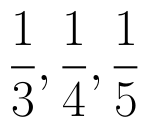and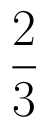. What is the probability that atmost one of them solve the problem?  (View Answer Video)

Question 5 :  Three numbers are selected at random (without replacement ) from first six positive integers. Let X denote the largest of the three numbers obtained. Find the probability distribution of X. Also, find the mean and variance of the distribution.  (View Answer Video)

### Matrices

Question 1 : Compute: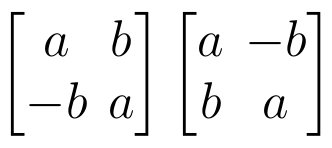. (View Answer Video)

Question 2 : Compute: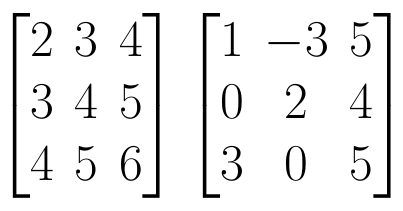. (View Answer Video)

Question 3 : Compute: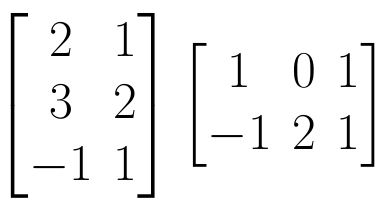. (View Answer Video)

Question 4 : Compute: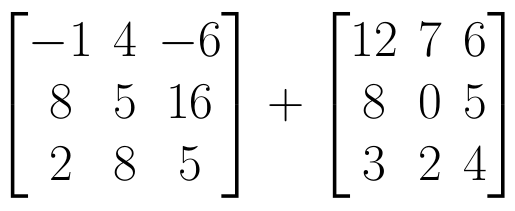. (View Answer Video)

Question 5 : If for any square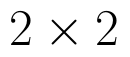matrix A,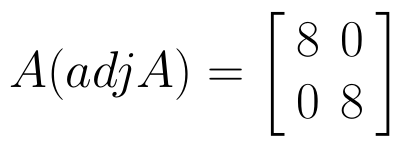then write the value of |A|. (View Answer Video)

### Three Dimensional Geometry

Question 1 : The equation of a line AB is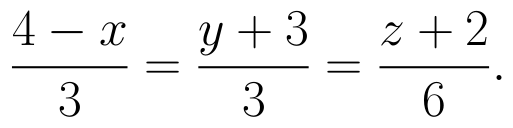Write the direction cosines of a line parallel to the above line. (View Answer Video)

Question 2 : Find the co-ordinates of the point where the line through the points A(3, 4, 1) and B(5, 1, 6) crosses the XY-plane. Also find angle which this line makes with the XZ-plane. (View Answer Video)

Question 3 : If a line has direction ratios 2, -1, -2, then what are its direction cosines? (View Answer Video)

Question 4 : Find the equation of the plane passing through the line of intersection of the planes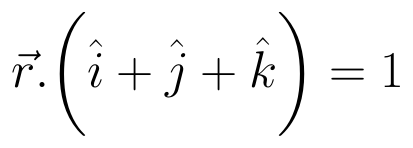and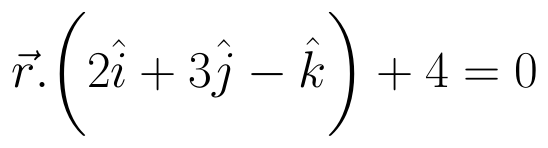which is perpendicular to the plane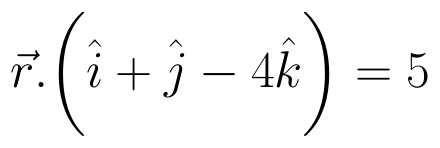. (View Answer Video)

Question 5 : Find the distance of the point (3, 4, 5) from the plane x + y + z = 2 measured parallel to the line 2x = y = z. (View Answer Video)

### Application of Derivatives

Question 1 : What is the maximum value of the function sin x+ cos x? (View Answer Video)

Question 2 : The normal at the point (1, 1) on the curve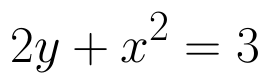is; (View Answer Video)

Question 3 : The slope of the normal to the curve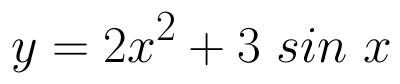at x = 0 is: (View Answer Video)

Question 4 : The total cost C(x) (in Rs) associated with the production of 'x' units of an item is given by :
C (x)=0.005x3-0.02x2+30x+5000.
Find the marginal cost when 3 units are produced, where by marginal cost we mean the instantaneous rate of change of total cost at any level of output. (View Answer Video)

Question 5 : The point on the curve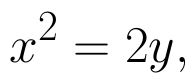which is nearest to the point (0, 5) is :